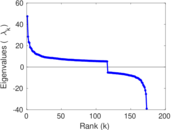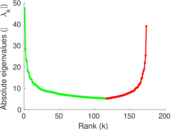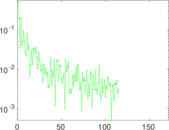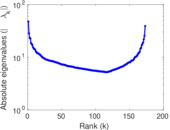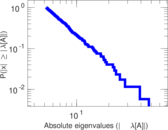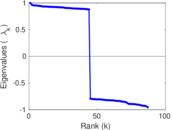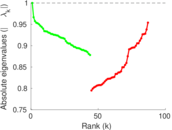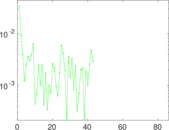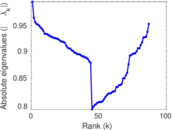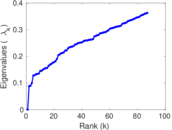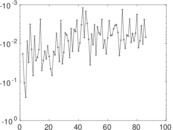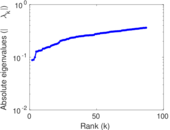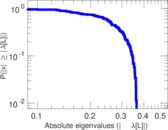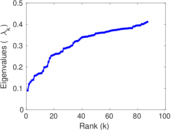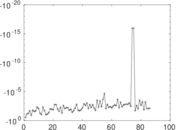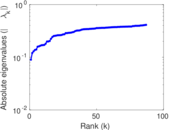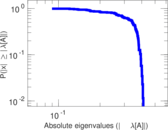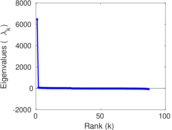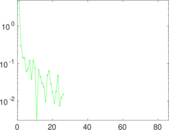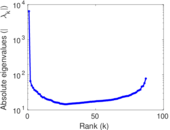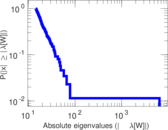Route views

This is the undirected network of autonomous systems of the Internet connected with each other. Nodes are autonomous systems (AS), and edges denote communitation. The network contains loops.

 Code AS Internal name as20000102 Name Route views Data source http://snap.stanford.edu/data/as.html AvailabilityDataset is available for download Consistency checkDataset passed all tests Category Computer network Node meaning Autonomous system Edge meaning Communication Network format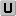Unipartite, undirected Edge typeUnweighted, no multiple edges Loops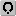Contains loops Orientation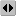Is not directed, but the underlying data is

Statistics

 Size n = 6,474 Volume m = 13,895 Loop count l = 1,323 Wedge count s = 2,059,364 Claw count z = 674,974,421 Cross count x = 212,651,094,228 Triangle count t = 6,584 Square count q = 288,840 4-Tour count T4 = 10,573,320 Maximum degree dmax = 1,459 Average degree d = 4.292 55 Fill p = 0.000 662 943 Size of LCC N = 6,474 Diameter δ = 9 50-Percentile effective diameter δ0.5 = 3.148 22 90-Percentile effective diameter δ0.9 = 4.449 21 Median distance δM = 4 Mean distance δm = 3.666 86 Gini coefficient G = 0.608 189 Balanced inequality ratio P = 0.273 480 Relative edge distribution entropy Her = 0.853 888 Power law exponent γ = 2.336 32 Tail power law exponent γt = 2.111 00 Tail power law exponent with p γ3 = 2.111 00 p-value p = 0.291 000 Degree assortativity ρ = −0.181 755 Degree assortativity p-value pρ = 1.098 82 × 10−185 Clustering coefficient c = 0.009 591 31 Spectral norm α = 47.476 6 Algebraic connectivity a = 0.088 030 8 Spectral separation |λ1[A] / λ2[A]| = 1.214 21 Non-bipartivity bA = 0.176 419 Normalized non-bipartivity bN = 0.045 798 5 Algebraic non-bipartivity χ = 0.089 770 8 Spectral bipartite frustration bK = 0.005 228 29 Controllability C = 3,605 Relative controllability Cr = 0.556 843

Plots

Fruchterman–Reingold graph drawing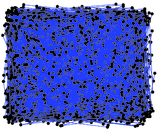Degree distribution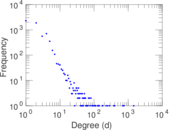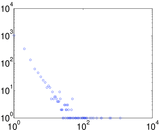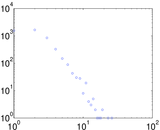Cumulative degree distribution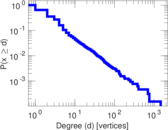Lorenz curve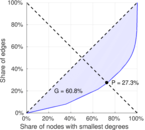Spectral distribution of the adjacency matrix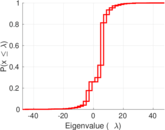Spectral distribution of the normalized adjacency matrix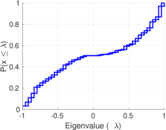Spectral distribution of the Laplacian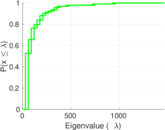Spectral graph drawing based on the adjacency matrix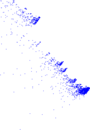Spectral graph drawing based on the Laplacian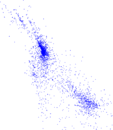Spectral graph drawing based on the normalized adjacency matrix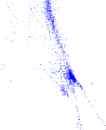Degree assortativity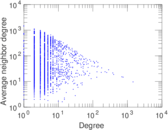Zipf plot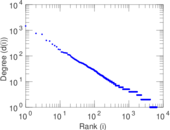Hop distribution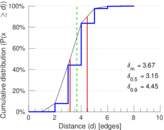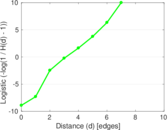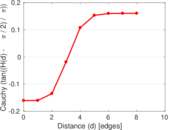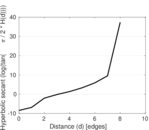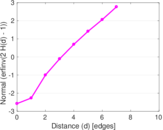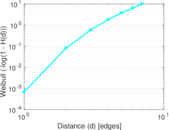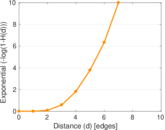Double Laplacian graph drawing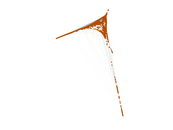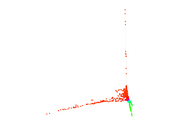Delaunay graph drawing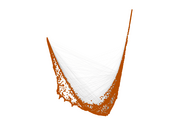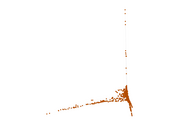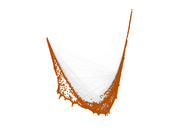Clustering coefficient distribution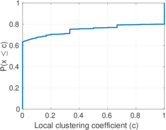Average neighbor degree distribution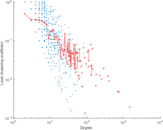SynGraphy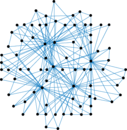Matrix decompositions plots